Hostname: page-component-594f858ff7-jtv8x Total loading time: 0 Render date: 2023-06-06T21:08:56.468Z Has data issue: false Feature Flags: { "corePageComponentGetUserInfoFromSharedSession": false, "coreDisableEcommerce": false, "corePageComponentUseShareaholicInsteadOfAddThis": true, "coreDisableSocialShare": false, "useRatesEcommerce": true } hasContentIssue false

## Abstract

Jeśmanowicz conjectured that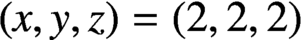$(x,y,z)=(2,2,2)$ is the only positive integer solution of the equation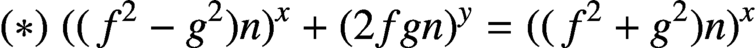$(*)\; ((\kern1.5pt f^2-g^2)n)^x+(2fgn)^y=((\kern1.5pt f^2+g^2)n)^x$ , where n is a positive integer and f, g are positive integers such that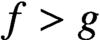$f>g$ ,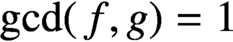$\gcd (\kern1.5pt f,g)=1$ and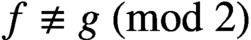$f \not \equiv g\pmod 2$ . Using Baker’s method, we prove that: (i) if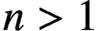$n>1$ ,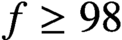$f \ge 98$ and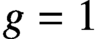$g=1$ , then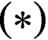$(*)$ has no positive integer solutions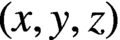$(x,y,z)$ with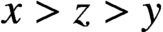$x>z>y$ ; and (ii) if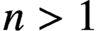$n>1$ ,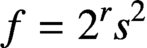$f=2^rs^2$ and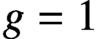$g=1$ , where r, s are positive integers satisfying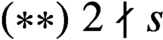$(**)\; 2 \nmid s$ and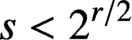$s<2^{r/2}$ , then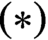$(*)$ has no positive integer solutions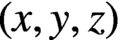$(x,y,z)$ with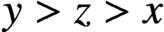$y>z>x$ . Thus, Jeśmanowicz’ conjecture is true if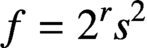$f=2^rs^2$ and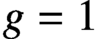$g=1$ , where r, s are positive integers satisfying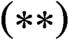$(**)$ .

## MSC classification

Type
Research Article
Information
© 2020 Australian Mathematical Publishing Association Inc.

## Access options

Get access to the full version of this content by using one of the access options below. (Log in options will check for institutional or personal access. Content may require purchase if you do not have access.)

## References

Bennett, M. A., Ellenberg, J. S. and Ng, N. C., ‘The Diophantine equation${A}^4+{2}^{\delta }{B}^2={C}^n$ ’, Int. J. Number Theory 6 (2010), 311338.CrossRefGoogle Scholar
Jeśmanowicz, L., ‘Several remarks on Pythagorean numbers’, Wiad. Mat. 1 (1955/1956), 196202 (in Polish).Google Scholar
Laurent, M., ‘Linear forms in two logarithms and interpolation determinants II’, Acta Arith. 133 (2008), 325348.CrossRefGoogle Scholar
Le, M.-H., ‘A note on Jeśmanowicz’ conjecture concerning Pythagorean triples’, Bull. Aust. Math. Soc. 59 (1999), 477480.CrossRefGoogle Scholar
Le, M.-H., Scott, R. and Styer, R., ‘A survey on the ternary purely exponential Diophantine equation${a}^x+{b}^y={c}^z$ ’, Surv. Math. Appl. 14 (2019), 109140.Google Scholar
Lu, W.-D., ‘On the Pythagorean numbers$4{n}^2-1$ ,$4n$ and$4{n}^2+1$ ’, J. Sichuan Univ. Nat. Sci. Ed. 5 (1959), 3942 (in Chinese).Google Scholar
Miyazaki, T., ‘A remark on Jeśmanowicz’ conjecture for the non-coprimality case’, Acta Math. Sin. Engl. Ser. 31 (2015), 12551260.CrossRefGoogle Scholar
Zhang, X.-W. and Zhang, W.-P., ‘The exponential Diophantine equation${(({2}^{2m}-1)n)}^x+{({2}^{m+1}n)}^y={(({2}^{2m}+1)n)}^z$ ’, Bull. Math. Soc. Sci. Math. Roumanie (N.S.) 57 (2014), 337344.Google Scholar